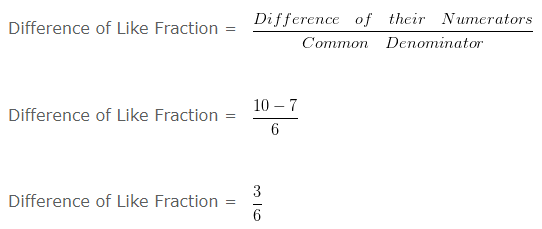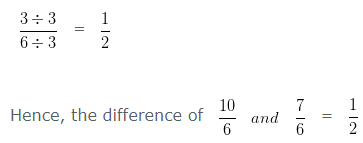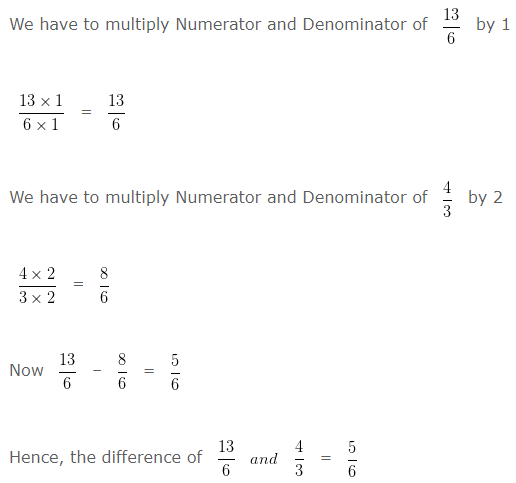# Subtraction of Like Fractions | Subtraction of Unlike Fractions

Subtraction of Like Fractions – To subtracts Like Fractions, we subtract the numerators and write the difference over the same denominator.

Subtraction of Unlike Fractions – To subtracts Unlike Fractions, first we have to change the Unlike Fractions, into equivalent Like Fractions, and then subtract the two equivalent Like Fractions

## Subtraction of Like Fractions Examples

QUESTION – 1

Find the difference of 10/6 and 7/6 and reduce to its lowest term.

EXPLANATIONOn simplifying further,

HCF of 3 and 6 is 3

Dividing both Numerator and Denominator by their HCF i.e, 3## Subtraction of Unlike Fractions Examples

QUESTION – 1

Find the difference of 13/6 and 4/3.

EXPLANATION

To find the difference of Unlike Fraction

Firstly, we have to change the Unlike Fraction into equivalent Like Fraction, and then subtract the two equivalent Like Fraction

LCM of 6 and 3 is 6

Converting the Unlike Fraction into Equivalent Like Fraction